# Bayesian Hierarchical Model¶

Oct. 23, 2018 $\newcommand{\bs}{\boldsymbol}$ $\newcommand{\argmin}{\underset{\bs{#1}}{\text{arg min}}\,}$ $\newcommand{\argmax}{\underset{\bs{#1}}{\text{arg max}}\,}$ $\newcommand{\tr}{^{\top}}$ $\newcommand{\norm}{\left|\left|\,#1\,\right|\right|}$ $\newcommand{\given}{\,|\,}$

In my previous post, I talked about ways to deal with clustering effect using machine learning techniques. Namely I encoded the clusters using a dummy variable, and used techniques such as support vector regression to predict the outcome. Today I will be talking about a more sophisticated way to model this situation, using a Bayesian linear mixed model.

For a fixed group $j$, we first propose a data model,

$$p(y_{j}^{(i)} \given \bs{x}_j^{(i)}, \bs{\beta}_j, \sigma^2) \tag{1}$$

where $\bs{x}_j^{(i)}$ is a $p\times 1$ vector of predictors for observation $i$ in group $j$, and $\sigma^2$ captures the variability of the model. The data model is dependent on the nature of the problem. For example, if $y_j^{(i)}$ is a continuous response, we often use the normal model,

$$y_{j}^{(i)} \given \bs{x}_j^{(i)}, \bs{\beta}_j, \sigma^2 \overset{i.i.d.}{\sim} N(\bs{\beta}_j\tr \bs{x}_j^{(i)}, \sigma^2).\tag{2}$$

For each group $j$, we combine all response $y^{(1)}_j,..., y^{(n_j)}_j$ into a single vector $\bs{Y}_j$ and combine the predictors $\bs{x}^{(1)}_j,..., \bs{x}^{(n_j)}_j$ into an $n_j \times p$ design matrix $\bs{X}_j$. We assume that the within-group response $y_j^{(i)}$ are independent; hence,

$$\bs{Y}_j \sim \mathcal{N} (\bs{X}_j \bs{\beta}_j, \sigma^2 \bs{I}), \quad j=1,...,m,$$

and the between-group data vectors $\bs{Y}_1,..., \bs{Y}_m$ are conditionally independent given the parameters $\bs{\beta}_1,..., \bs{\beta}_m$ and $\sigma^2$.

The normal hierarchical regression model describes the heterogeneity among different groups using a multivariate normal distribution:

$$\{\bs{\beta}_j\} \overset{i.i.d.}{\sim} \mathcal{N}(\bs{\theta}, \bs{\Sigma}), \quad j=1,..., m.$$

Here, note that the covariance matrix $\bs{\Sigma}$ is not required to be a diagonal, implying mutual dependence among the regression coefficients. The situation can be summarized by the following figure.Using a different representation, this is equivalent to

\begin{aligned} \bs{\beta}_j &= \bs{\theta} + \bs{\gamma}_j \\ \{\bs{\gamma}_j\} & \overset{i.i.d.}{\sim} \mathcal{N}(\bs{0}, \bs{\Sigma}), \,\, j=1,...,m. \end{aligned} \tag{3}

Combining (2) and (3) gives the linear mixed model:

\begin{aligned} y_j^{(i)} &= \bs{\theta}\tr \bs{x}_j^{(i)} + \bs{\gamma}_j\tr \bs{x}_j^{(i)} + \epsilon_{j}^{(i)}. \\ \{\bs{\gamma}_j\} & \overset{i.i.d.}{\sim} \mathcal{N}(\bs{0}, \bs{\Sigma}), \quad j=1,...,m. \\ \{\epsilon_j^{(i)}\} & \overset{i.i.d.}{\sim} N(0, \sigma^2), \quad i=1,..., n_j, \, \text{ for all fixed } j. \end{aligned} \tag{3}

In this parametrization, $\bs{\theta}$ is referred to as the fixed effect, as it is constant across all groups. The parameter $\bs{\gamma}_j$ are called random effects, as they are unique across different groups. One thing to note is that although the features corresponding to the fixed and random effects are the same in (3), they do not have to be. A more general model is

$$y_j^{(i)} = \bs{\theta}\tr \bs{x}_j^{(i)} + \bs{\gamma}_j\tr \bs{z}_i^{(j)} + \epsilon_j^{(i)}, \tag{4}$$

where $\bs{x}_j^{(i)}$ and $\bs{z}_j^{(i)}$ could contain different sets of predictors. In particular, $\bs{x}_j^{(i)}$ usually contain group-specific predictors where as $\bs{z}_j^{(i)}$ are the predictors that are used to distinguish among groups.

## Bayesian Spatial Regression Model¶

We express the vectorized spatial regression model below.

$$\bs{Y} = \bs{X}\bs{\theta} + \bs{Z}\bs{b} + \bs{\epsilon}. \tag{5}$$

Here, $\bs{Y} \in \mathbb{R}^n$ is the response vector where $n=\sum n_j$ is the total number of training examples. $\bs{X} \in \mathbb{R}^{n\times p}$ is the design matrix for the data. The vector $\bs{\theta} \in \mathbb{R}^p$ is the fixed effect, and the vector $\bs{b} \in \mathbb{R}^m$ is the spatial random effect. Finally, the matrix $\bs{Z} \in \mathbb{R}^{n\times m}$ is the location matrix. The $i$th row of the location matrix $\bs{Z}$ is the one-hot encoder for the geographical location of the $i$th training example.

Furthermore, the Bayesian normal model assumes the following prior distributions.

\begin{aligned} \bs{\epsilon} &\sim \mathcal{N}(\bs{0}, \sigma^{2} \bs{I}) \\ \bs{\theta} &\sim \mathcal{N}(\bs{0}, \bs{R}) \\ \sigma^{-2} &\sim \text{Gamma}(a_{\sigma}, b_{\sigma}) \\ \bs{b} &\sim \text{ICAR}(\sigma^2\tau^2, \rho) \\ \tau^{-2} &\sim \text{Gamma}(a_{\tau}, b_{\tau}). \end{aligned}\tag{6}

Note that here we assume that the response $\bs{Y}$ follows a multivariate normal distribution. However, this is not always appropriate when the response is binary or counts.

## ICAR Model¶

Before preceding, we will talk about the reason behind using the Intrinsic Conditional Autoregressive Model (ICAR) model to capture the spatial random effect $\bs{b}$. The vector $\bs{b}$ is said to follow an $\text{ICAR}(\sigma^2\tau^2, \rho)$ model if

$$\bs{b} \sim \mathcal{N}(\bs{0},\, \sigma^2\tau^2(\bs{D}-\rho\bs{W})^{-1}), \tag{7}$$

where $\rho$ controls the strength of spatial dependence, and $\bs{W} = (W_{i,j})$ is the neighborhood matrix, such that

$$W_{i,j} = \begin{cases} 1 & \text{ if } i \text{ neighbors } j \\ 0 & \text{ otherwise. } \end{cases}$$

Traditionally, neighbors are usually geographical units that border each other. Hence $\bs{W}$ is a sparse matrix. And $\bs{D} = \text{diag}(D_{i,i})$ is a diagonal matrix such that

$$D_{i,i} = \sum_j W_{i,j}.$$

In other words, $\bs{D}$ is a matrix whose $i$th diagonal entry counts the number of neightbors for location $i$.

For example, suppose we have a 3x3 grid labeled as follows.The adjacency matrix in this case can be visualized as follows.

In :
library(Matrix)
library(dplyr)

#     group = list of n^2 locations arranged in nxn grid
n = length(group)
# I and J are lists of indices of adjacent cells
I = list()
J = list()
k=1
for (i in 1:n){
for (j in 1:n){
if (abs(group[i]-group[j]) %in% c(1, 4, 5, 6)){
I[[k]] <- i
J[[k]] <- j
k = k+1
}
}
}
W <- sparseMatrix(unlist(I), unlist(J), x=1)
d <- W %>% as.matrix() %>% rowSums()
D <- sparseMatrix(1:n, 1:n, x=d)
return(list('W'=W, 'D'=D))
}

In :
neighbors <- neighbor.load(1:9)
W <- neighbors$W D <- neighbors$D
print(W)
print(D)

9 x 9 sparse Matrix of class "dgCMatrix"

[1,] . 1 . . 1 1 1 . .
[2,] 1 . 1 . . 1 1 1 .
[3,] . 1 . 1 . . 1 1 1
[4,] . . 1 . 1 . . 1 1
[5,] 1 . . 1 . 1 . . 1
[6,] 1 1 . . 1 . 1 . .
[7,] 1 1 1 . . 1 . 1 .
[8,] . 1 1 1 . . 1 . 1
[9,] . . 1 1 1 . . 1 .
9 x 9 sparse Matrix of class "dgCMatrix"

[1,] 4 . . . . . . . .
[2,] . 5 . . . . . . .
[3,] . . 5 . . . . . .
[4,] . . . 4 . . . . .
[5,] . . . . 4 . . . .
[6,] . . . . . 4 . . .
[7,] . . . . . . 5 . .
[8,] . . . . . . . 5 .
[9,] . . . . . . . . 4


It turns out that the ICAR model has the following property:

$$b_i \given \bs{b}_{-i} \sim N\left(\frac{\rho\sum_{k=1}^n W_{k,i} b_k}{\sum_{k=1}^n W_{k,i}}, \frac{\sigma^2\tau^{2}}{\sum_{k=1}^n W_{k,i}}\right). \tag{8}$$

where $\bs{b}_{-i} = \{b_j \given j\neq i\}$. We see that the conditional mean of $b_i$ given all other components is a weighted mean of its neighbors. The variance is divided by the total number of neighbors of $b_i$. This structure is also known as the Gaussian Markov Random Field (GMRF).

From (6), we can write down the joint posterior distribution as follows.

\begin{aligned} p(\bs{\theta}, \sigma^{-2}, \tau^{-2}, \bs{b} \given \bs{X}, \bs{Y}) &\propto (\sigma^{-2})^{\frac{n}{2}} \text{exp}\left(\frac{-(\bs{Y}-\bs{X}\bs{\theta}-\bs{Z}\bs{b})\tr(\bs{Y}-\bs{X}\bs{\theta}-\bs{Z}\bs{b})}{2\sigma^{2}}\right) \\ & \quad \times \text{exp}\left(\frac{-\bs{\theta}\bs{R}^{-1}\bs{\theta}}{2}\right)\\ & \quad \times (\sigma^{-2})^{a_{\sigma}-1} \text{exp}(-\sigma^{-2} b_{\sigma}) \\ & \quad \times (\tau^{-2})^{a_{\tau}-1} \text{exp}(-\tau^{-2} b_{\tau}) \\ & \quad \times (\sigma^{-2}\tau^{-2})^{\frac{n}{2}} \text{exp}\left(\frac{-\bs{b}\tr(\bs{D}-\rho\bs{W})\bs{b}}{2\sigma^2\tau^2}\right). \end{aligned} \tag{9}

## Full Conditionals¶

Since the joint distribution in (9) is unbreakable into marginal distributions, we need to find the full conditionals. We start with the parameter $\bs{\theta}$. Letting $\bs{Y}^* = \bs{Y}-\bs{Z}\bs{b}$, we have

\begin{aligned} p(\bs{\theta} \given \text{else}) &\propto \text{exp}\left(-\frac{1}{2\sigma^{2}}\left((\bs{Y}^* - \bs{X}\bs{\theta})\tr(\bs{Y}^*-\bs{X}\bs{\theta}) + \sigma^{2}\bs{\theta}\tr \bs{R}^{-1}\bs{\theta}\right) \right)\\ &\propto \text{exp}\left(-\frac{1}{2\sigma^{2}}\left(\bs{\theta}\tr(\bs{X}\tr\bs{X} + \sigma^{2}\bs{R}^{-1})\bs{\theta}) - 2{\bs{Y}^*}\tr \bs{X}\bs{\theta}\right)\right) \end{aligned} \tag{10}

We compare this kernel to the kernel of a multivariate normal $\bs{Z} \sim \mathcal{N}(\bs{\mu}, \sigma^{2}\bs{\Sigma})$ which has the form

\begin{aligned} f_{\bs{Z}} &\propto \text{exp}\left(-\frac{1}{2\sigma^{2}}(\bs{Z}-\bs{\mu})\tr \bs{\Sigma}^{-1}(\bs{Z}-\bs{\mu})\right) \\ &= \text{exp}\left(-\frac{1}{2\sigma^{2}}(\bs{Z}\tr \bs{\Sigma}^{-1}\bs{Z} - 2\bs{Z}\tr \bs{\Sigma}^{-1}\bs{\mu} + \bs{\mu}\tr\bs{\Sigma}^{-1}\bs{\mu})\right) \\ & \propto \text{exp}\left(-\frac{1}{2\sigma^{2}}(\bs{Z}\tr\bs{\Sigma}^{-1}\bs{Z}-2\bs{\mu}\tr\bs{\Sigma}^{-1}\bs{Z}) \right). \end{aligned} \tag{11}

Comparing (10) with (11), we conclude that

$$\bs{\theta} \given \text{else} \sim \mathcal{N}\left((\bs{X}\tr\bs{X} + \sigma^{2}\bs{R}^{-1})^{-1} \bs{X}\tr(\bs{Y}-\bs{Z}\bs{b}), \quad \sigma^{2}(\bs{X}\tr\bs{X}+\sigma^{2}\bs{R}^{-1})^{-1}\right). \tag{12}$$

Next we examine the full conditional distribution of the precision parameter $\sigma^{-2}$.

$$p(\sigma^{-2} \given \text{else}) \propto (\sigma^{-2})^{\frac{n}{2}+a_{\sigma}-1} \text{exp}\left(-\sigma^{-2}\left(b_{\sigma} + \frac{(\bs{Y}-\bs{X}\bs{\theta}-\bs{Z}\bs{b})\tr(\bs{Y}-\bs{X}\bs{\theta}-\bs{Z}\bs{b})}{2} + \frac{\bs{b}\tr(\bs{D}-\rho\bs{W})\bs{b}}{2\tau^2}\right) \right)$$

Immediately we recognize that

$$\sigma^{-2} \given \text{else} \sim \text{Gamma}\left(a_{\sigma}+\frac{n}{2},\quad b_{\sigma} + \frac{(\bs{Y}-\bs{X}\bs{\theta}-\bs{Z}\bs{b})\tr(\bs{Y}-\bs{X}\bs{\theta}-\bs{Z}\bs{b})}{2} + \frac{\bs{b}\tr(\bs{D}-\rho\bs{W})\bs{b}}{2\tau^2} \right) \tag{13}$$

Similarly, we have

$$\tau^{-2} \given \text{else} \sim \text{Gamma}\left(a_{\tau}+\frac{n}{2},\quad b_{\tau} + \frac{\bs{b}\tr(\bs{D}-\rho\bs{W})\bs{b}}{2\sigma^2}\right) \tag{14}$$

Finally, using symmetry from (12), we have

$$\bs{b} \given \text{else} \sim \mathcal{N}\left((\bs{Z}\tr\bs{Z} + \tau^{-2}(\bs{D}-\rho\bs{W}))^{-1} \bs{Z}\tr(\bs{Y}-\bs{X}\bs{\theta}), \quad \sigma^{2}(\bs{Z}\tr\bs{Z} + \tau^{-2}(\bs{D}-\rho\bs{W}))^{-1}\right). \tag{15}$$

## Gibbs Sampling¶

Before we implement the MCMC algorithm, we will talk about efficient ways to block sample from a multivariate normal distribution. Consider the problem of sampling from $\bs{X}$ whose pdf has the form

$$p(\bs{X}) \propto \text{exp}\left(-\frac{1}{2}\bs{X}\tr\bs{A}\bs{X} + \bs{a}\tr\bs{X}\right).$$

Comparing this to (11), we conclude that

$$\bs{X} \sim \mathcal{N}(\bs{A}^{-1}\bs{a}, \bs{A}^{-1}).$$

To obtain a sample from $\bs{X}$, we use the following algorithm:

Algorithm 1 Sampling from multivariate normal

• Steps to obtain a sample from $\mathcal{N}(\bs{0}, \bs{A}^{-1})$:
• Sample $\bs{z}\sim \mathcal{N}(\bs{0}, \bs{I})$. This is computationally fast.
• Compute the Choleskey decomposition of $\bs{A}=\bs{L}\bs{L}\tr$.
• Solve $\bs{L}\tr \bs{y} = \bs{z}$ for $\bs{y}$ by forward substitution.
• $\bs{y} = (\bs{L}\tr)^{-1}\bs{z}$ is a sample from $\mathcal{N}(\bs{0}, \bs{A}^{-1})$.
• Steps to compute $\bs{A}^{-1}\bs{a}$:
• Solve $L\bs{v} = \bs{a}$ for $\bs{v}$ by backward substitution.
• The solution is $\bs{v} = \bs{L}^{-1} \bs{a}$.
• Solve $\bs{L}\tr \bs{u} = \bs{v}$ for $\bs{u}$.
• $\bs{u} = \bs{A}^{-1}\bs{a}$.
• $\bs{x} = \bs{u} + \bs{y}$ is a sample from $\mathcal{N}(\bs{A}^{-1}\bs{a}, \bs{A}^{-1})$.

We implement in R as follows.

In :
sample.normal <- function(a, A){
#   sampling from N(Q^-1*b, Q^-1)
n <- length(a)
Lt <- chol(A) # chol(A) gives upper triangular part
y <- solve(Lt, rnorm(n, 0, 1), sparse=TRUE)
v <- solve(t(Lt), a, sparse=TRUE)
u <- solve(Lt, v, sparse=TRUE)
u + y
}

In :
# Test this function
A <- cbind(c(1, 0.5), c(0.5, 1))
a <- c(1, 3)
sample.normal(a, A)

1. -1.15889320090909
2. 3.62680161987532

We are now ready for gibbs sampling. Recall our full conditionals are:

\left\{ \begin{aligned} \bs{\theta} \given \text{else} &\sim \mathcal{N}\left((\bs{X}\tr\bs{X} + \sigma^{2}\bs{R}^{-1})^{-1} \bs{X}\tr(\bs{Y}-\bs{Z}\bs{b}), \quad \sigma^{2}(\bs{X}\tr\bs{X}+\sigma^{2}\bs{R}^{-1})^{-1}\right) \\ \sigma^{-2} \given \text{else} &\sim \text{Gamma}\left(a_{\sigma}+\frac{n}{2},\quad b_{\sigma} + \frac{(\bs{Y}-\bs{X}\bs{\theta}-\bs{Z}\bs{b})\tr(\bs{Y}-\bs{X}\bs{\theta}-\bs{Z}\bs{b})}{2} + \frac{\bs{b}\tr(\bs{D}-\rho\bs{W})\bs{b}}{2\tau^2} \right) \\ \tau^{-2} \given \text{else} &\sim \text{Gamma}\left(a_{\tau}+\frac{n}{2},\quad b_{\tau} + \frac{\bs{b}\tr(\bs{D}-\rho\bs{W})\bs{b}}{2\sigma^2}\right) \\ \bs{b} \given \text{else} &\sim \mathcal{N}\left((\bs{Z}\tr\bs{Z} + \tau^{-2}(\bs{D}-\rho\bs{W}))^{-1} \bs{Z}\tr(\bs{Y}-\bs{X}\bs{\theta}), \quad \sigma^{2}(\bs{Z}\tr\bs{Z} + \tau^{-2}(\bs{D}-\rho\bs{W}))^{-1}\right). \end{aligned} \right.\tag{16}

In :
library(Matrix)

model.gibs <- function(X, Y, Z, W, D, rho, R, b, sig2, tau2, as, bs, at, bt, iterations, plot=TRUE) {

#     Gibbs sampling [theta -> b -> sigma^2 -> tau^2]
#     -----------
#     Parameters:
#     -----------
#     b, sig2, tau2 = initialized values for the chain
#     R = prior covariance matrix for theta
#     as, bs, at, bt = parameters for the gamma distribution
#     X, Y, Z = design matrix, label vector, and location matrix
#     W, D, rho = the spatial random effects

n <- length(Y)
m <- length(b)
I <- sparseMatrix(1:n, 1:n, x=1)
DW <- D-rho*W

# Creating saving devices
theta.MCMC <- matrix(0, nrow=iterations, ncol=2)
sig2.MCMC <- rep(0, iterations)
tau2.MCMC <- rep(0, iterations)
b.MCMC <- matrix(0, nrow=iterations, ncol=m)

for (i in 1:iterations){
# Sampling theta
A <- (t(X)%*%X + sig2*solve(R))/sig2
a <- t(X)%*%(Y-Z%*%b)/sig2
theta <- sample.normal(a, A)
theta.MCMC[i,] <- as.vector(theta)

# Sampling b
Q <- DW/tau2
A <- (t(Z)%*%Z + Q)/sig2
a <- t(Z)%*%(Y-X%*%theta)/sig2
b <- sample.normal(a, A)
b.MCMC[i,] <- as.vector(b)

# Sampling sigma^2
alpha <- as + n/2
beta <- as.vector(bs + sum((Y-X%*%theta-Z%*%b)^2)/2 + t(b)%*%Q%*%b/2)
sig2 <- 1/rgamma(1, alpha, beta)
sig2.MCMC[i] <- sig2

# Sampling tau^2
alpha <- at + n/2
beta <- as.vector(bt + t(b)%*%DW%*%b/(2*sig2))
tau2 <- 1/rgamma(1, alpha, beta)
tau2.MCMC[i] <- tau2
}

if(plot==TRUE) {
options(repr.plot.width=9, repr.plot.height=4)
par(mfrow=c(2, 2), mar=c(4, 4, 1, 1))
plot(theta.MCMC[,1], ylab="", xlab="", main=bquote(theta))
plot(b.MCMC[,1], ylab="", xlab="", main=bquote(b))
plot(sig2.MCMC, ylab="", xlab="", main=bquote(sigma^2))
plot(tau2.MCMC, ylab="", xlab="", main=bquote(tau^2))
}

return(list("theta"=theta.MCMC, "sig2"=sig2.MCMC, "tau2"=tau2.MCMC, "b"=b.MCMC))
}


## Simulation Study¶

For a simulation study, let's generate a univariate dataset according to (3):

\begin{aligned} y_j^{(i)} &= \bs{\theta}\tr \bs{x}_j^{(i)} + \bs{\gamma}_j\tr \bs{x}_j^{(i)} + \epsilon_{j}^{(i)}. \\ \{\bs{\gamma}_j\} & \overset{i.i.d.}{\sim} \mathcal{N}(\bs{0}, \bs{\Sigma}), \quad j=1,...,m. \\ \{\epsilon_j^{(i)}\} & \overset{i.i.d.}{\sim} N(0, \sigma^2), \quad i=1,..., n_j, \, \text{ for all fixed } j. \end{aligned}

In :
library(mvtnorm)

data.load <- function(m=9, theta=rep(1,p), seed=2, sigma=20, n=100, p=2) {

#     parameters:
#     -----------
#     sigma = variance for the noise
#     theta = fixed effect
#     m = number of regions
#     n = number of samples per region
#     p = number of columns of design matrix

set.seed(seed)

# Generating a random covariance matrix
A <- matrix(runif(p^2), ncol=p)
Sigma <- t(A) %*% A %>% as.matrix()

# Generating X
X <- rep(1, n*m)
for (i in 1:(p-1)){
X <- cbind(X, runif(n*m, 0, 100))
}

# Generating response Y
Y <- rep(0, n*m)
gamma <- rmvnorm(m, rep(0,p), Sigma) %>% as.matrix()

for (j in 1:m){
n.lower <- 1+n*(j-1)
n.upper <- n*j
X.j <- X[n.lower:n.upper, ]
gamma.j <- gamma[j,] %>% as.vector()
Y[n.lower:n.upper] <- X.j%*%theta + X.j%*%gamma.j + rnorm(n, 0, sigma)
}

loc <- list()
for (j in 1:m){
loc[[j]] <- rep(j, n)
}
X <- cbind(X, Y, unlist(loc))
return(X)
}


Let's generate data from 9 locations, each containing 100 data points.

In :
library(data.table)
mydata.df <- mydata %>% as.data.table()
names(mydata.df) <- c('X0', 'X1', 'Y', 'Group')

In :
head(mydata.df)

X0X1YGroup
1 94.38393 69.1711051
1 94.34750 67.0392681
1 12.91590 24.3377891
1 83.34488 7.5603571
1 46.80185 24.7440001
1 54.99837 6.4793351

Let's plot the data.

In :
# Plotting all data combined
options(repr.plot.width=4, repr.plot.height=4)
plot(mydata.df[,X1], mydata.df[,Y], xlab='X', ylab='Y', main='Combined')
abline(lm(Y ~ X1, data=mydata.df), col='red')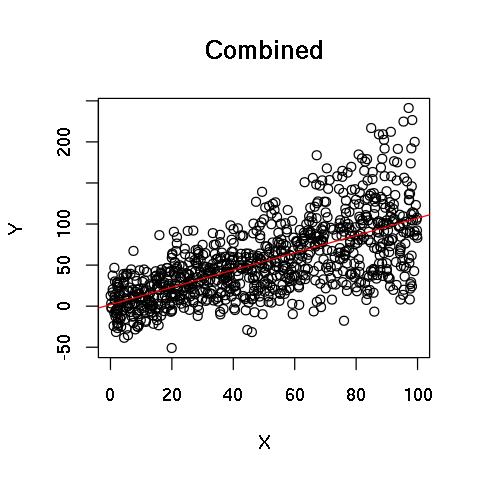We see that without including a spatial random effect, a simple linear model lacks the flexibility to learn the data. This can be seen by plotting each location separately.

In :
# Plotting separately
options(repr.plot.width=9, repr.plot.height=9)
par(mfrow=c(3, 3), mar=c(4, 4, 1, 1))

for (i in 1:9){
plot(mydata.df[Group==i, X1], mydata.df[Group==i, Y],
ylim=c(-100, 200), xlab='X', ylab='Y', main=paste('Group',i))
abline(lm(mydata.df[Group==i, Y] ~ mydata.df[Group==i, X1]), col='red')
}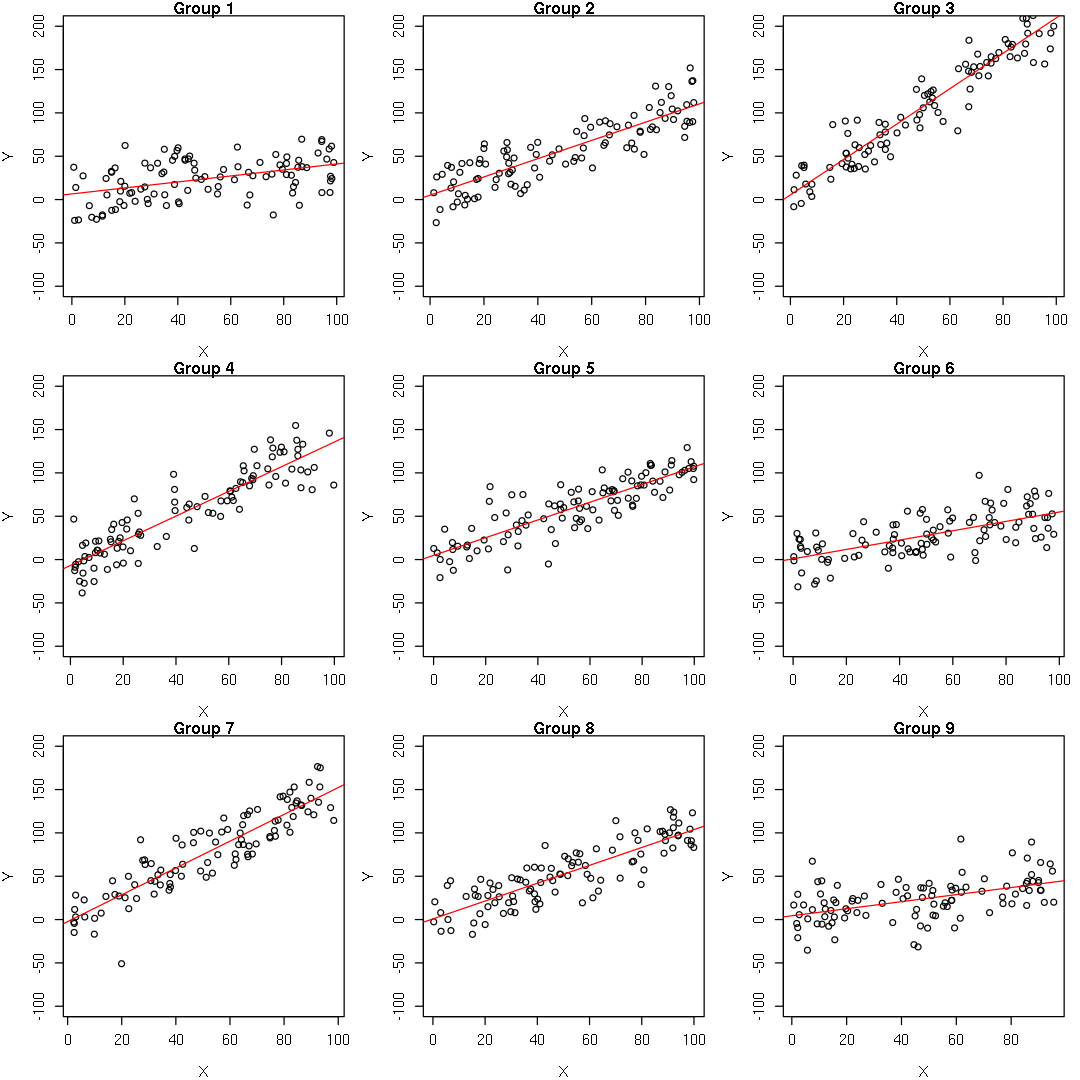Indeed, within each cluster, there is a strong linear relationship. To run our model, the last thing we need is the location matrix $\bs{Z}$. We do this by one-hot encoding the last column of the data frame.

In :
# Returns location matrix from numeric vector
one_hot <- function(vector) {
m <- unique(vector) %>% length()
n <- length(vector)
I <- diag(m)
Z <- matrix(0, n, m)
for (i in 1:n) {
Z[i,] <- I[vector[i],] %>% as.vector()
}
return(Z)
}


We are now ready to build our model.

In :
# Building data matrices X and Y
X <- mydata[,c(1, 2)]
Y <- mydata[,3]
Z <- one_hot(mydata[,4])

In :
# Starting the model
params <- model.gibs(X, Y, Z, W, D, 1, R=diag(rep(10, 2)), b=rep(1, 9),
sig2=10, tau2=10, as=10, bs=10, at=10, bt=10, iterations=5000, plot=TRUE)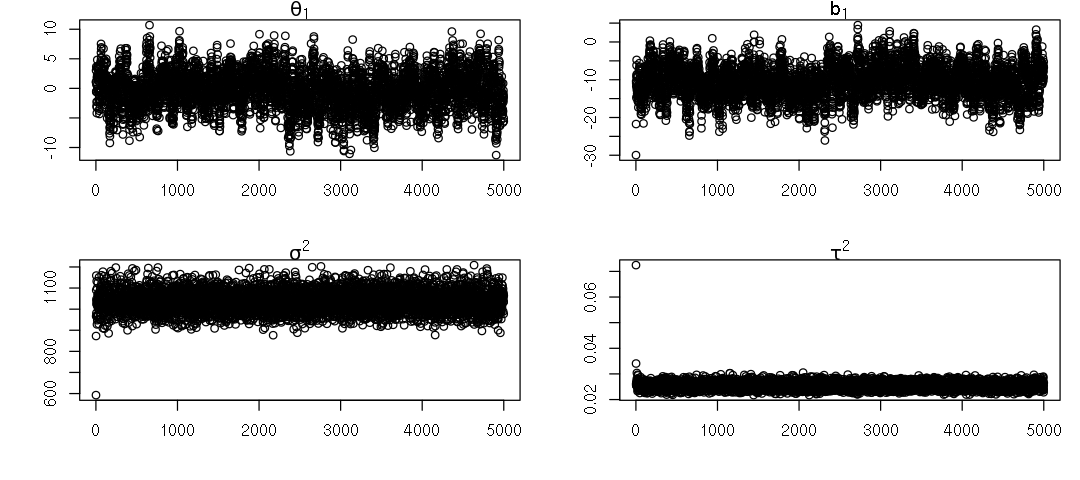In :
# Finding the posterior estimate of our parameters :D
theta.est <- params$theta[2500:5000, ] %>% colMeans() b.est <- params$b[2500:5000, ] %>% colMeans()
tau2.est<- params$tau2[2500:5000] %>% mean() sig2.est <- params$sig2[2500:5000] %>% mean()

print(paste(c('Theta is:', theta.est), collapse=" "))
print(paste(c('b is:', b.est), collapse=" "))
print(paste(c('tau2 is:', tau2.est), collapse=" "))
print(paste(c('sig2 is:', sig2.est), collapse=" "))

 "Theta is: -0.649151756548812 1.04679070018715"
 "b is: -9.97592191353502 4.5413974864405 22.2153409980448 7.49568585916764 -0.70917608476754 -8.7728210493963 10.0261387355896 5.43856981142533 -4.32814314393123"
 "tau2 is: 0.0254396115572093"
 "sig2 is: 1037.80649997605"

In :
# Visualize first 4 components of b
options(repr.plot.width=9, repr.plot.height=4)
par(mfrow=c(2, 2), mar=c(4, 4, 1, 1))
plot(params$b[,1], ylab="", xlab="", main=bquote(b)) plot(params$b[,2], ylab="", xlab="", main=bquote(b))
plot(params$b[,3], ylab="", xlab="", main=bquote(b)) plot(params$b[,4], ylab="", xlab="", main=bquote(b))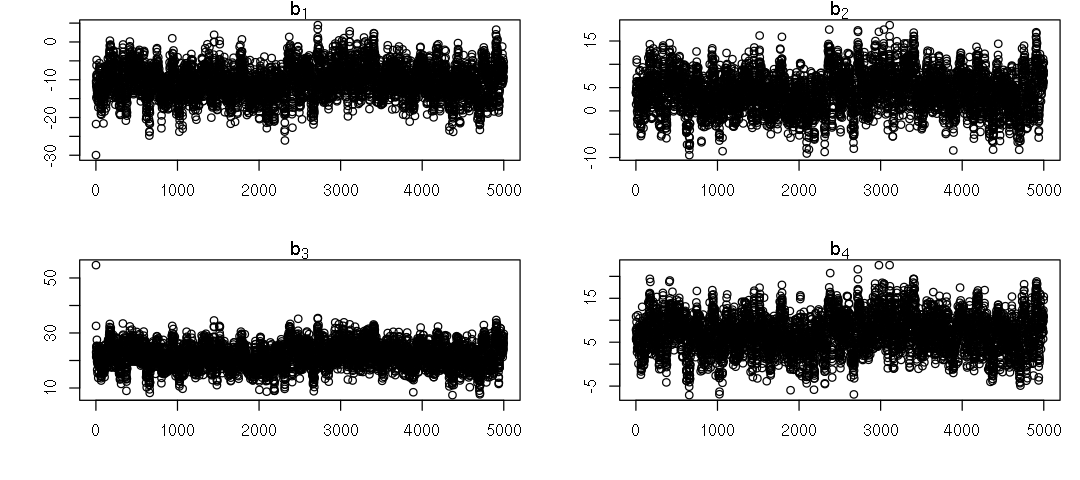Let's see how our model is doing by adding one more column as the predicted values.

In :
Y.pred <- X %*% theta.est + Z %*% b.est
mydata.df <- cbind(mydata.df, Y.pred)

In :
head(mydata.df)

X0X1YGroupV1
1 94.38393 69.1711051 88.175151
1 94.34750 67.0392681 88.137008
1 12.91590 24.3377891 2.895168
1 83.34488 7.5603571 76.619573
1 46.80185 24.7440001 38.366669
1 54.99837 6.4793351 46.946713
In :
# Plotting the prediction!
options(repr.plot.width=9, repr.plot.height=9)
par(mfrow=c(3, 3), mar=c(4, 4, 1, 1))

for (i in 1:9){
plot(mydata.df[Group==i, X1], mydata.df[Group==i, Y],
ylim=c(-100, 200), xlab='X', ylab='Y', main=paste('Group',i))
points(mydata.df[Group==i, X1], mydata.df[Group==i, V1], ylim=c(-100, 200), col='red')
}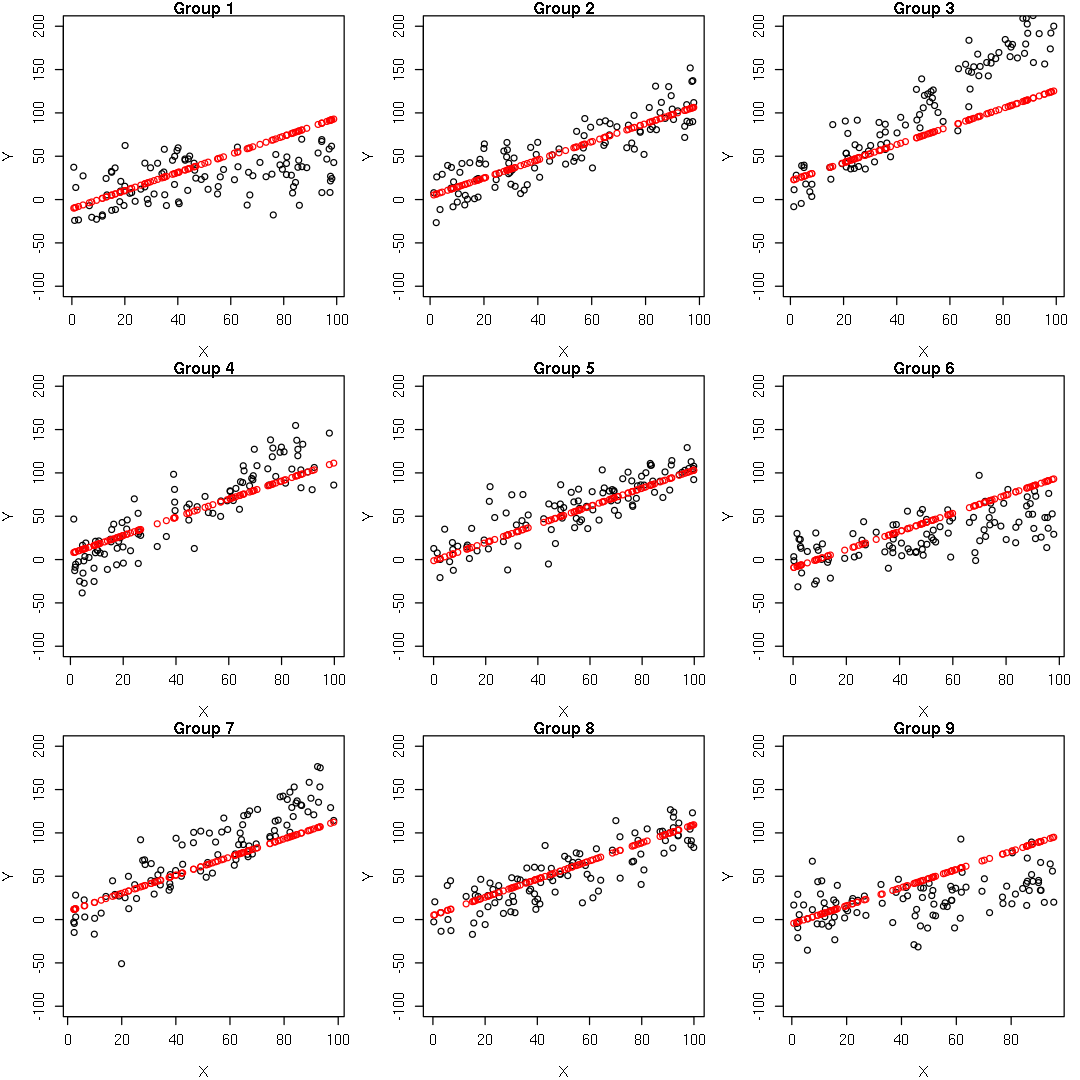This is not bad for such a simple model. Stay tuned for more advanced models!